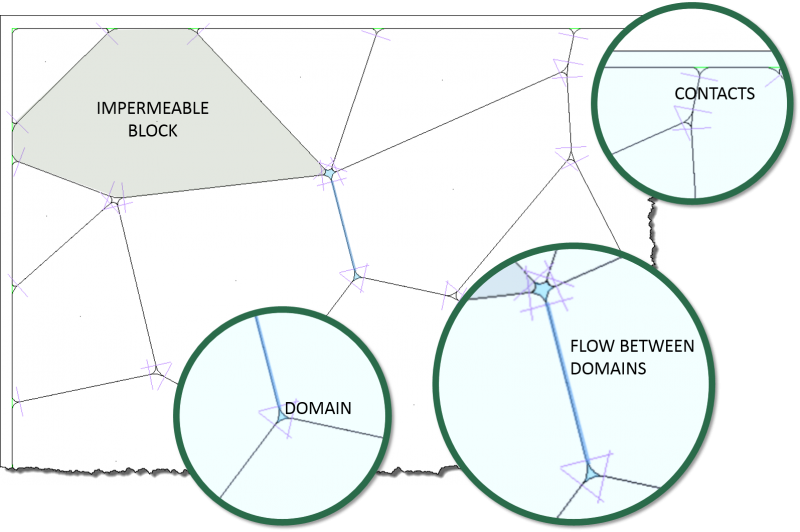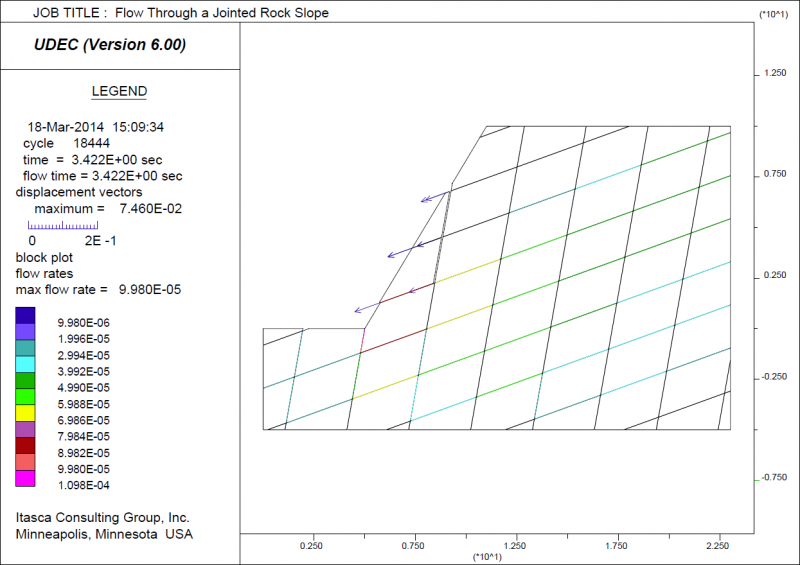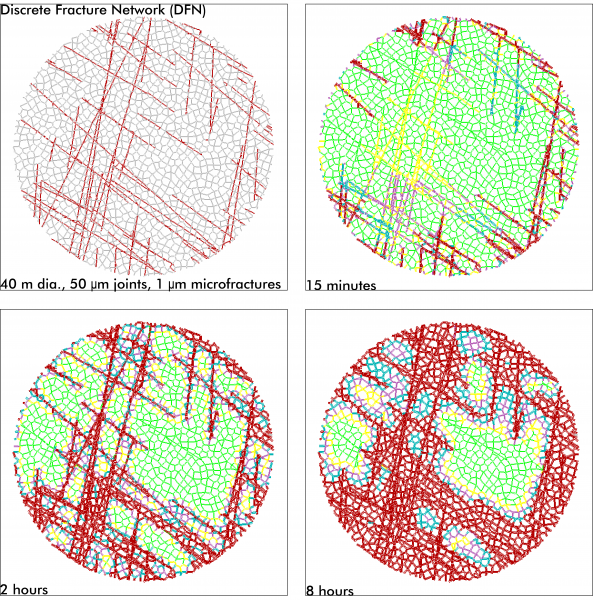## Fluid Flow in Joints in UDEC

UDEC has the capability to perform the analysis of fluid flow through the fractures and voids of a system of impermeable blocks. Steady-state pore pressures can be assigned to zones within deformable blocks and boundary conditions may be applied in terms of fluid pressures or by defining an impervious boundary. A porous medium can be defined around the UDEC block model to simulate a regional flow field.

The numerical implementation for fluid flow in UDEC makes use of the domain structure. For a closely packed system, there is a network of domains, each of which is assumed to be filled with fluid at uniform pressure and which communicates with its neighbors through contacts. Domains are separated by the contact points, which are the points at which the forces of mechanical interaction between blocks are applied. Because deformable blocks are discretized into a mesh of triangular elements, gridpoints may exist not only at the vertices of the block, but also along the edges. A contact point will be placed wherever a gridpoint meets an edge or a gridpoint of another block. Therefore, the degree of refinement of the numerical representation of the flow network is linked to the mechanical discretization adopted. In the absence of gravity, a uniform fluid pressure is assumed to exist within each domain. For problems with gravity, the pressure is assumed to vary linearly according to the hydrostatic gradient, and the domain pressure is defined as the value at the center of the domain. Flow is governed by the pressure differential between adjacent domains.The fluid-flow calculation can also be run either coupled or uncoupled with the mechanical stress calculation. A fully coupled mechanical-hydraulic analysis is performed in which fracture conductivity is dependent on deformation, and conversely, joint fluid pressures affect the mechanical computations. Both confined flow and flow with a free surface can be modeled with this formulation. Flow is idealized as laminar viscous flow between parallel plates. A visco-plastic flow model is also available to simulate flow of cement grout in the joints. The joint permeability relation can be modified and one-way thermal-hydraulic coupling of flow in joints, in which temperature variations induce changes in the viscosity and density of water, can also be simulated.

The fluid-calculation modes available include the following.

• Compressible Liquid, Steady-State Flow
• Compressible Liquid, Transient Flow
• Incompressible Liquid, Transient Flow
• Compressible Gas, Transient Flow
• Two-Phase Fluid, Transient Flow
##### Some Examples

The following UDEC model shows the effect of raising the water table of a jointed slope from 6 m to 10 m. The fluid flow-rate and rock-block displacement vectors are shown.The following is an example of a circular domain model consisting of both microcracks (Voronoi with very low permeability) within the rock matrix and larger-scale, moderately permeable joints. With the outer domain pressurized, the flow of water can be traced over time.